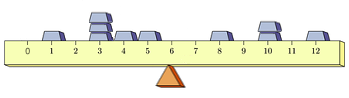Expected Value and Center of Mass

One might wonder why the function that gives the probabilities of the various outcomes associated with a random variable is called a "probability mass function".

Suppose several lead weights of various masses are glued at different positions along the top of a ruler of negligible mass and width. This collection of masses is balanced on a small triangular prism that functions as a fulcrum, as shown in the picture below.While one could envision the above as 10 equal-sized masses, with three of these at position 3 on the ruler and two of these at position 10 -- to better demonstrate the connection between the probability mass function for a random variable and the physics of balancing masses, let us instead see this as 7 masses: five masses of size $m$ at positions 1, 4, 5, 8, and 12; a mass double that size (i.e., $2m$) at position 10; and another mass triple that size (i.e., $3m$) at position 3.

Viewing things in this way, we can then define a function $f(x)$ that for any position $x$ on the ruler, outputs the fraction of the total mass located at that position.

This function $f(x)$ can easily be described by a table, as shown below

$$\begin{array}{|r|c|c|c|c|c|c|c|}\hline x & 1 & 3 & 4 & 5 & 8 & 10 & 12\\\hline f(x) & 0.1 & 0.3 & 0.1 & 0.1 & 0.1 & 0.2 & 0.1\\\hline \end{array}$$

Physics tells us that if the masses are to balance atop the triangular prism without tipping to one side or the other, the collective torque of the masses to the left of the triangular prism must be equal in magnitude to the collective torque of the masses to the right of the triangle, but be opposite in direction (i.e., sign).

Recall that torque is the product of the force on an object and the distance to the pivot point in question (i.e., the triangular prism). Further, recall that physics also tells us that a mass of $m$ will exert a force of $mg$ on the surface upon which it rests, where $g$ is the acceleration due to gravity.

Let $M$ be the total of all of the masses involved, and $\mu$ be the position of the triangular prism. Noting that the mass at position $x_i$ is given by $M \cdot f(x_i)$, and consequently the force exerted by this mass on the ruler is $[M \cdot f(x_i)] \cdot g$, we can see that the torque $\tau$ contributed by the mass at position $x_i$ must be given by the product $$\tau = (x_i - \mu) \cdot [M \cdot f(x_i)] \cdot g$$

The sum of all of these torques needs to be zero if the ruler is to balance on the triangular prism. So in this case, $$\begin{array}{rcl} 0 &=&(1-\mu) \cdot [M \cdot (0.1)] \cdot g \\ && {} + (3-\mu) \cdot [M \cdot (0.3)] \cdot g \\ && {} + (4-\mu) \cdot [M \cdot (0.1)] \cdot g \\ && {} + (5-\mu) \cdot [M \cdot (0.1)] \cdot g \\ && {} + (8-\mu) \cdot [M \cdot (0.1)] \cdot g \\ && {} + (10-\mu) \cdot [M \cdot (0.2)] \cdot g \\ && {} + (12-\mu) \cdot [M \cdot (0.1)] \cdot g \end{array}$$ Dividing both sides by $M$ and $g$, and solving for $\mu$, we can find exactly where to place the triangular prism to balance the masses -- a position known as the center of mass. $$\begin{array}{rcl} \mu &=& (1)(0.1) + (3)(0.3) + (4)(0.1) + (5)(0.1) + (8)(0.1) + (10)(0.2) + (12)(0.1)\\ &=& 5.9 \end{array}$$

We can of course do this in general for any number of masses. The results are similar -- assuming a finite number of masses at positions $x_1,x_2,\ldots, x_n$, the center of mass is given by $\mu$ where: $$\mu = x_1 \cdot f(x_1) + x_2 \cdot f(x_2) + \cdots + x_n \cdot f(x_n)$$

Presuming $S$ is the set of all positions where there are masses (i.e., $S = \{x_1,x_2,\ldots,x_n\}$), we can write this in an even tighter way: $$\mu = \sum_{x \in S} [x \cdot f(x)]$$

Recall the formula for the expected value of a random variable, X: $$E(X) = \sum_{x \in S} [x \cdot P(x)]$$ In addition to the obvious parallels between the formula for the center of mass and that for the expected value, one should also note that just as $0 \le P(x) \le 1$ for any outcome $x \in S$ -- it must also be the case that $0 \le f(x) \le 1$ (since $f(x)$ is a "fraction of the total mass" a single mass of the collection represents)..

With such a strong connection between $P(x)$ and $f(x)$, the name "probability mass function" for $P(x)$ seems kinda' appropriate now, doesn't it!